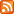### research!rsc

#### Thoughts and links about programming, by Russ Cox# Random Hash Functions Posted on Sunday, April 1, 2012.

A hash function for a particular hash table should always be deterministic, right? At least, that's what I thought until a few weeks ago, when I was able to fix a performance problem by calling rand inside a hash function.

A hash table is only as good as its hash function, which ideally satisfies two properties for any key pair k1, k2:

1. If k1 == k2, hash(k1) == hash(k2).
2. If k1 != k2, it should be likely that hash(k1) != hash(k2).

Normally, following rule 1 would prohibit the use of random bits while computing the hash, because if you pass in the same key again, you'd use different random bits and get a different hash value. That's why the fact that I got to call rand in a hash function is so surprising.

If the hash function violates rule 1, your hash table just breaks: you can't find things you put in, because you are looking in the wrong places. If the hash function satisfies rule 1 but violates rule 2 (for example, “return 42”), the hash table will be slow due to the large number of hash collisions. You'll still be able to find the things you put in, but you might as well be using a list.

The phrasing of rule 1 is very important. It is not sufficient to say simply “hash(k1) == hash(k1)”, because that does not take into account the definition of equality of keys. If you are building a hash table with case-insensitive, case-preserving string keys, then “HELLO” and “hello” need to hash to the same value. In fact, “hash(k1) == hash(k1)” is not even strictly necessary. How could it not be necessary? By reversing rule 1, hash(k1) and hash(k1) can be unequal if k1 != k1, that is, if k1 does not equal itself.

How can that happen? It happens if k1 is the floating-point value NaN (not-a-number), which by convention is not equal to anything, not even itself.

Okay, but why bother? Well, remember rule 2. Since NaN != NaN, it should be likely that hash(NaN) != hash(NaN), or else the hash table will have bad performance. This is very strange: the same input is hashed twice, and we're supposed to (at least be likely to) return different hash values. Since the inputs are identical, we need a source of external entropy, like rand.

What if you don't? You get hash tables that don't perform very well if someone can manage to trick you into storing things under NaN repeatedly:

```\$ cat nan.py
#!/usr/bin/python
import timeit
def build(n):
m = {}
for i in range(n):
m[float("nan")] = 1
n = 1
for i in range(20):
print "%6d %10.6f" % (n, timeit.timeit('build('+str(n)+')',
'from __main__ import build', number=1))
n *= 2

\$ python nan.py
1   0.000006
2   0.000004
4   0.000004
8   0.000008
16   0.000011
32   0.000028
64   0.000072
128   0.000239
256   0.000840
512   0.003339
1024   0.012612
2048   0.050331
4096   0.200965
8192   1.032596
16384   4.657481
32768  22.758963
65536  91.899054
\$
```

The behavior here is quadratic: double the input size and the run time quadruples. You can run the equivalent Go program on the Go playground. It has the NaN fix and runs in linear time. (On the playground, wall time stands still, but you can see that it's executing in far less than 100s of seconds. Run it locally for actual timing.)

Now, you could argue that putting a NaN in a hash table is a dumb idea, and also that treating NaN != NaN in a hash table is also a dumb idea, and you'd be right on both counts.

But the alternatives are worse:

• If you define that NaN is equal to itself during hash key comparisons, now you have a second parallel definition of equality, to handle NaNs inside structs and so on, only used for map lookups. Languages typically have too many equality operators anyway; introducing a new one for this special case seems unwise.
• If you define that NaN cannot appear as a hash table key, then you have a similar problem: you need to build up logic to test for invalid keys such as NaNs inside structs or arrays, and then you have to deal with the fact that your hash table might return an error or throw an exception when inserting values under certain keys.

The most consistent thing to do is to accept the implications of NaN != NaN: m[NaN] = 1 always creates a new hash table element (since the key is unequal to any existing entry), reading m[NaN] never finds any data (same reason), and iterating over the hash table yields each of the inserted NaN entries.

Behaviors surrounding NaN are always surprising, but if NaN != NaN elsewhere, the least surprising thing you can do is make your hash tables respect that. To do that well, it needs to be likely that hash(NaN) != hash(NaN). And you probably already have a custom floating-point hash function so that +0 and −0 are treated as the same value. Go ahead, call rand for NaN.

(Note: this is different from the hash table performance problem that was circulating in December 2011. In that case, the predictability of collisions on ordinary data was solved by making each different table use a randomly chosen hash function; there's no randomness inside the function itself.)